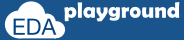# SVA Methods

## \$rose

```\$rose(boolean expression or signal name)
```

returns true if the least significant bit of the expression changed to 1. Otherwise, it returns false.

```sequence seq_rose;
@(posedge clk) \$rose(a);
endsequence
```

Sequence seq_rose checks that the signal "a" transitions to a value of 1 on every positive edge of the clock. If the transition does not occur, the assertion will fail.

## \$fell

```\$fell(boolean expression or signal name)
```

returns true if the least significant bit of the expression changed to 0. Otherwise, it returns false.

```sequence seq_fell;
@(posedge clk) \$fell(a);
endsequence
```

Sequence seq_fell checks that the signal "a" transitions to a value of 0 on every positive edge of the clock. If the transition does not occur, the assertion will fail.

## \$stable

```\$stable(boolean expression or signal name)
```

returns true if the value of the expression did not change. Otherwise, it returns false.

```sequence seq_stable;
@(posedge clk) \$stable(a);
endsequence
```

Sequence seq_stable checks that the signal "a" is stable on every positive edge of the clock. If there is any transition occurs, the assertion will fail.

## \$past

```\$past(signal_name, number of clock cycles)
```

provides the value of the signal from the previous clock cycle.

Below Property checks that, in the given positive clock edge, if the “b” is high, then 2 cycles before that, a was high.

```property p;
@(posedge clk) b |-> (\$past(a,2) == 1);
endproperty
a: assert property(p);
```

Execute the above code on## \$past construct with clock gating

The \$past construct can be used with a gating signal. on a given clock edge, the gating signal has to be true even before checking for the consequent condition.

```\$past (signal_name, number of clock cycles, gating signal)
```

Below Property checks that, in the given positive clock edge, if the “b” is high, then 2 cycles before that, a was high only if the gating signal "c' is valid on any given positive edge of the clock.

```Property p;
@(posedge clk) b |-> (\$past(a,2,c) == 1);
endproperty
a: assert property(p);
```

Execute the above code on## Built-in system functions

• \$onehot(expression)
• checks that only one bit of the expression can be high on any given clock edge.
• \$onehot0(expression)
• checks only one bit of the expression can be high or none of the bits can be high on any given clock edge.
• \$isunknown(expression)
• checks if any bit of the expression is X or Z.
• \$countones(expression)
• counts the number of bits that are high in a vector.

```a_1: assert property( @(posedge clk) \$onehot(state) );
a_2: assert property( @(posedge clk) \$onehot0(state) );
a_3: assert property( @(posedge clk) \$isunknown(bus) ) ;
a_4: assert property( @(posedge clk) \$countones(bus)> 1 );
```

Assert statement a_1 checks that the bit vector "state" is one-hot.
Assert statement a_2 checks that the bit vector "state" is zero one-hot.
Assert statement a_3 checks if any bit of the vector "bus" is X or Z.
Assert statement a_4 checks that the number of ones in the vector "bus" is greater than one.